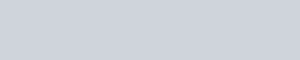# Formula to convert kVA to kW, single-phase, two-phase and three-phase

We will thoroughly analyze the formula from kVA to kW, clearly defining what each variable that composes it means, how they interrelate and what is the difference between them.

Mas información:

## The formula used for the conversion of kVA to kW is as follows:With this formula you can manually convert from kVA to kW, however if you want to convert automatically you can use the kVA to kW

## Definitions of each variable, kVA, kW and power factor:

kVA: Commonly called apparent power and designated with the letter “S”, the power in kVA is the total power of an equipment or inductive system, what does this mean ?, that the power in kVA will only be present in equipment that has components that require some type of induction, such as: motors, generators, transformers, etc.

Being a bit more technical we can say that the kVA is produced when the voltage and current are not in phase, therefore the KVA will be different to the KW, this only happens when the voltage and current are out of phase, it should be keep in mind that the KVA will always be higher than the KW, this leads to another question that are then the kW ?.

kW: This power is called real power and is designated with the letter “P”, is the power that actually does the work and is present in all electrical equipment whether inductive or resistive, what does it mean? This power is used in all electrical appliances regardless of whether they have motors, resistors, electronics, etc. to perform the work of heating, lighting, movement, pumping, etc. This power is always lower than the kVA. Then the last question that is the power factor and how it relates to the kVA and kW ?.

Power factor : The power factor defines how efficiently electricity is used. Which indicates the total power in kVA how much is actually used in kW.

The power factor is the ratio (phase) of the current and voltage in the AC electrical distribution systems. Under ideal conditions, the current and voltage are “in phase” and the power factor is “100%”. If there are inductive loads (motors), a power factor lower than 100% (typically 80 to 90%) may occur. Some typical values ​​of power factor for equipment are presented in this table .

The low power factor, speaking electrically, causes a greater current to flow in the power distribution lines to deliver a given number of kilowatts to an electrical load.

## Relationship and difference between kVA, kW and Fp:

In other words, the kVA can not be without the kW but the latter if they can be without the kVA, which indicates that in alternating current (AC) there are the kVA and kW while in direct current (DC) there are only the kW, this occurs because in AC there is the power factor (FP), while in direct current there is no FP or is equal to 1.

From the above we can deduce that the difference between kVA and kW is the famous power factor that depending on the current (AC or DC) and electrical equipment fluctuates between zero (0) and one (1).

Now if we replace in the formula the value of power factor equal to 1, we can realize that the kW are converted into kVA (KW = kVAx1), the value of 1 only occurs in DC circuits and purely resistive loads (AC) , in reality these last ones are not common because all the real charges AC have something of inductive.

It must be kept in mind that it is the same formula for monophasic, biphasic and three-phase systems.

[kkstarratings]